# Maths# Maths# Maths# Maths

## Maths for Allied Health

#### The maths of human movement

All Allied Health courses involve the basic maths of human movement.

You will develop an understanding of Biomechanics which is the study of how force is applied to the human body and the body applies force to various objects. It involves understanding and applying mathematical formulae (algebra) to show the relationship between variables.

We will introduce you to:

• the measurement systems and common abbreviations used
• vectors and scalars
• how forces are measured in human movement.

Have you studied Year 12 Physics or Advanced Mathematics?

If you have not, there is a self-test called the Numeracy Success Indicator (NSI), which you can complete. It will help identify what you still need to learn and how to go about learning it.

### Measuring human movement

The following points are a ready reference. Check you understand them and if not browse through some basic biomechanics text books at 612.76 in the library.

#### Measurement systems and abbreviations

Displacement can be linear or angular.s or d = linear displacement, the difference between the final position and the initial position and includes the direction taken

s or d refer to linear displacement only

θ refers to angular displacement that rotates around a central point

Velocity, the magnitude of the change in displacement of an object can be linear or angular.

v = linear velocity, is the change in displacement over the change in time

ω= angular velocity of a rotation around a central point

#### Acceleration, the change in velocity over a period of time.

a = linear acceleration, is the change in velocity per unit of time t. If it increases the acceleration is positive. If it decreases the acceleration is negative.

α = angular acceleration is around a central point

#### Abbreviations and common knowledge in Kinematics

∆ = change

Time is measured in seconds (s)

Length is measured in meters (m)

#### Linear motion and forces

Linear motion uses this acceleration formula a = F/m where:

m = mass, a measurement of inertia, using kilograms (kg) as a unit of measure.

F = Force is measured in (N) newtons.

N (a newton) is measured in kg/metres/s2.

W = Weight, a downward directed force, is defined by the gravitational pull of the earth on an object. It  is measured in (N) newtons.

g = the gravitational constant ie 9.8 metres per second2

m = W/g   A 1kg mass has a weight of 9.8 Newtons (N).

Linear momentum (P), is the product of a moving body's mass (m) and linear velocity (v) where:

P = mv measured in  units of kg/metres/s2 , in a particular direction

#### Angular momentum and forces

Angular momentum (A) uses a similar formula  A = Iω   where I = moment of inertia and ω = angular velocity.

Moment of inertia offers resistance to change in rotary motion. Its value relies on the object's mass (m) and its distance  from the centre of rotation (r).

I =  mxr2.

### Vectors and scalars

Velocity, acceleration, force or displacement all have both magnitude and direction, therefore have vector quantities. If described by their magnitude alone they are termed scalars. Scalars can be added, subtracted, multiplied and divided. Vectors are treated geometrically using triangles and parallelograms to solve problems. Vectors are used to determine the velocity of movement while scalars only help to determine speed.• Calculating velocity means finding the change in displacement (m) over the time taken (s) while speed is measured by finding the distance covered over the time taken. Both velocity and speed are calculated on this Khan Academy video: Velocity and Speed
• There are also summary sheets on Geometry if you want more information.

#### Equations – linear vectors

When looking at movement, line vectors are used to determine the velocity, the acceleration and the displacement of the person or object moving. These are the basic equations to be used: There is some background information in the Measurement Systems and Common Abbreviations section.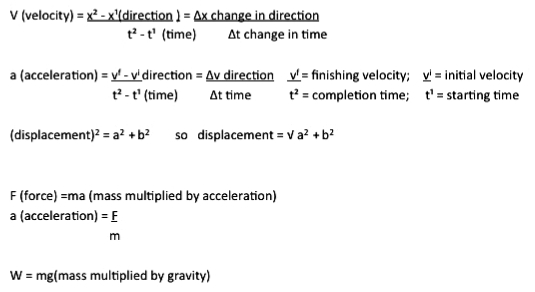#### Equations – angular vectors

Angular vectors, unlike linear vectors, do not use distance measurements. Instead they use the measures of angular motion: of degrees or radians. There are 360° in a full rotation of a circle. 1 radian ≈ 57.3° and 1 degree (1°) ≈ 0.0175 radians. The following equations are the basics needed to understand the introduction to using angular vectors.Angular velocity ω describes the speed of rotation and the orientation of the axis around which the object rotates.Fig 3. Angular vectors

### Forces in human movement & Newton's Laws

An important type of vector used to determine movement in Biomechanics is force. Force is a push or a pull that either produces, halts or changes the direction of the motion of an object. The forces to consider in biomechanics are: gravitational, contact, frictional, muscular, inertial, elastic, buoyant and electromagnetic. These forces have various effects:

If the magnitude of the force exceeds the magnitude of resistance of the body, there will be a change in motion. Inertia and friction need to be factored in. (For more information on this check the Measurement Systems and Common Abbreviations and Vectors and Scalars sections.)

#### Acceleration

Acceleration is vital in understanding how forces work.

#### Newton's laws of motion

Newton’s Three Laws of Motion are other important points to understand about force:

The First Law of Motion explains inertia

• where an object at rest remains at rest unless acted upon by an outside force;
• where an object in motion remains in motion at constant velocity unless acted upon by an outside force; and
• where an object in motion tends to move in a straight line unless acted upon by an outside force.The Second Law of Motion is the Law of Acceleration:

• The acceleration (a) of an object is directly proportional to the outside force applied to the object and inversely proportional to the object’s mass.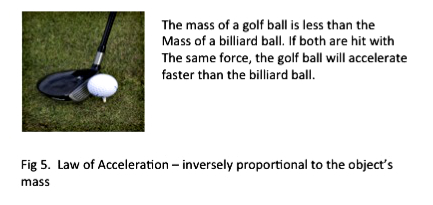Newton’s third law is the Law of Action- Reaction:

• Where for every action or force applied to an object, the object responds with an equal and opposite reaction. This applies to the forces exerted when walking on a surface.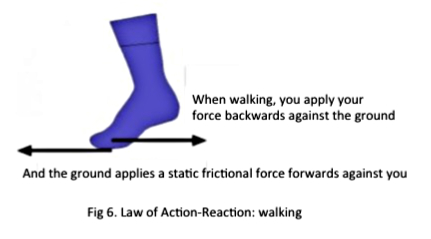When calculating forces in biomechanics of human movement, you will need to consider the application of these three laws of Newton and vectors.

## Statistics for Allied Health

#### Why do you need statistics skills?

When you have graduated from your allied health course, your daily practice will be guided by evidence found through research. Research relies heavily on statistics for evidence when testing a hypothesis. Having confidence in the pre-requisite Maths skills for understanding basic statistical analysis will help in completing certain assessments.

The following introduces you to some basic statistical concepts, the arithmetic and algebra used in basic statistics, how to interpret graphs and some statistical measured used in studying Allied Health subjects.

#### Test yourself and know where to get help

If you have not completed Advanced Maths in your final years of secondary education, you are encouraged to self-test your Maths foundation skills. Then check that you are confident with the following concepts in the next three sections.

There is more detailed information in the Statistics section of this guide If you identify a concept that you need help with you can find services at the page Where to go for help. There is also detailed statistics help on request from the Maths Skills program.

#### Arithmetic and Algebra for using statistics in Allied Health

The following is a list of underlying concepts that are often confused. Check the list to be sure you are familiar with the contents.In Allied Health research studies, factors can found have a positive (+ ) or negative (- ) relationship with each other. For example attitudes of long-term friends to certain issues such as abortion are more likely to be similar than to those of a stranger. This illustrates a positive relationship.

#### What does'equals' really mean?

The equals sign = means that both sides of an equation have the same value. Therefore both sides of an equation must be kept balanced. The balanced scales demonstrate this.Fig 1. Balanced scales

If something is added to or subtracted from one side, it should also be added to or subtracted from the other side. If one side is multiplied or divided by that number, the other side must also be multiplied or divided by that number.

#### Basic rules of operations & processes:

Adapted from Kranzler and Moursund (1995)

Addition and subtraction of positive and negative numbers

Rule 1: if a digit does not have a + or – sign, it is inferred it is a positive number

Rule 2: When adding up numbers with the same sign (+ or -) put that common sign as a prefix

Rule 3: When adding up numbers with a mixture of signs, add up the +ve numbers and add up the –ve numbers, then subtract the smaller sum from the larger sum with the sign of the larger sum as the prefix in the answer.

Rule 4: Subtracting a +ve number is the same as adding a –ve number.

Rule 5: Subtracting a –ve number results in a +ve answer.

Multiplication and Division of +ve and –ve numbers

Rule 6: Multiplying or dividing two –ves results in a positive answer.

Rule 7: Multiplying or dividing numbers with different signs gives a –ve answer

Rule 8: For a long list of numbers to be multiplied or divided, follow the rules 6  & 7,  and make the calculations in pairs

Fractions

Rule 9: A fraction symbolises a division of the number above the line (numerator) being divided by the number below the line (denominator)

Rule 10: Any number can be made a fraction by making a denominator of 1

Rule 11: To multiply fractions, multiply the numerators together and multiply the denominators together

Rule 12: To divide by a fraction, invert the fraction and continue as for Rule 11

Rule 13: To add or subtract a fraction, find the common denominator of both fractions then proceed

Decimals and percentages

Rule 14: a decimal indicates the fraction of 10, 100, 1000 and so on, when those numbers are denominators.

E.g., .2 =­ 2/10 .02 = 2/100

Rule 15: When a number is less than one, write a 0 before the decimal point 0.2

Rule 16:  Rounding off is needed when converting some fractions to decimals because there are endless digits in the answer.

If 1.414 is rounded off to 2 decimal places it becomes 1.41 because

if the final digit  is less than (< ) 5 that digit is discarded.

However 1.416 rounded off to 2 decimal places becomes 1.42 because if the final digit is greater than (>) 5 the second last digit is increased by 1

If the final digit is exactly 5, e.g., 1.425 it is discarded then should the second last digit of the number be even number, is kept e.g., 1.42.

However if it is odd, e.g., 1.475 it rounded up by 1 e.g., 1.48.

Examples (even): 1.425 ---> 1.42 (odd): 1.475 ----->  1.48

Exponents, Powers and Roots

An exponent is written like this 32. This means 3 is multiplied by itself or squared (2) 3x3 = 9. If the exponent is greater than 3, e.g., (35), the number is said, in this example, to be raised to the power of 5. See the Table 1 for more details

Table 1. Exponents and Powers

 3 exponent Expression Expanded form Answer 30 Zero power of 3 1 31 3 to the power of 1 3 3 32 3 squared 3x3 9 33 3 cubed 3x3x3 27 34 3 to the power of 4 3X3X3X3 81

A square root is written like this √9. The square root of a number is the number, that when squared, equals the radicand (number inside the √). This means √3x3 = 3. or in other words, 9 results from 3 being multiplied by 3. [see Table 2.]

Table 2. Roots

 Roots Expression Expanded form Answer √9 Square root of 9 √3x3 3 3√27 Cubed Root of 27 √3X3X3 3 4√81 Fourth Root of 81 √3x3x3x3 3

Order of Computation – BODMAS Rule

Rule 17:  In an equation where there is a long list of processes, order the steps by following this rule:

#### References

Kranzler, G., & Moursund, J. (1995). Statistics for the terrified. Englewood Cliffs, NJ Prentice Hall.

#### Interpreting graphs that represent test results

There are several types of graphs that can be used to represent data collected in research.

#### Histograms or Bar Graphs

Frequency shown is on the vertical axis. The values of the variables are represented on the horizontal axis. The height of each rectangle (bar) coming up from the horizontal axis is the frequency of occurrence of that value. There is an extra score one number below the bottom score in the range and one number above the top score in the range.Fig 2. Example of histogram. Adapted from Pallant, J. (2013). SPSS survival manual: A step by step guide to data analysis using IBM SPSS (5th ed.). Sydney, Melbourne, Auckland, London: Allen & Unwin.

#### Frequency polygon

This is another form of representation of frequency but the bar for each score is replaced with a point directly above each score on the horizontal axis. Then the points for each score are connected in sequence by straight lines. The line starts on the point 1 number below the lowest score and finishes one number above the highest score. These types of graphs can be overlaid to make comparisons between groups of test scores.Fig 3 Example of frequency polygon

#### Normal distribution curve

This is an expression of typical behaviour distribution, called normal probability and is symmetrical with a cluster about the mean. The normal distribution curve represents a smoothed normal histogram and the area between the curve and the horizontal axis represents all of the measurements in any distribution.

• 68% of all the scores are within +/- 1s of the mean
• 95% of all scores are within +/- 2s of the mean
• 99.7% of all the scores are within +/- 3s of the mean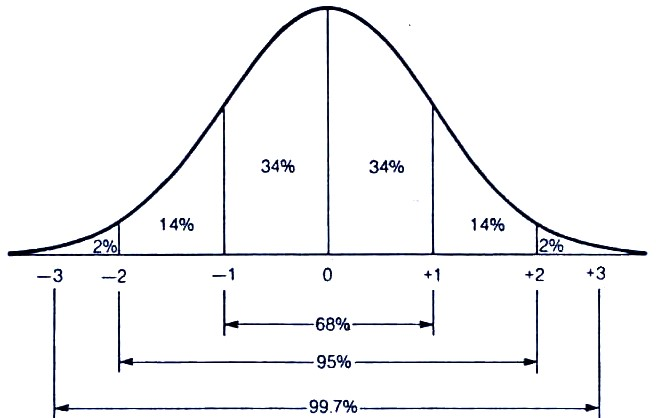Fig 4 Normal distribution curve

#### Kurtosis: Mesokurtic, Leptokurtic or Platykurtic distribution

The scores of smaller samples when plotted may not be symmetrical and instead produce a skewed curve.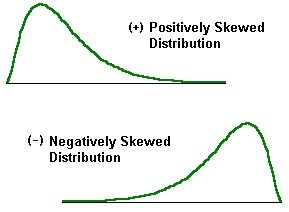Fig 5. Skewed distribution curves

The other results can be a kurtosis where the curve may be more peaked around the mean (Leptokurtic) or flattened (Platykurtic) around the mean. Leptocurtic curves indicate a data set which is clustered around the mean. Mesokurtic curves indicate a normally distributed data set. Platykurtic curves indicate a data set that is highly dispersed.

Kurtosis describes the flatness or pointedness of the curve in a graph. The other results have around the mean, a more peaked curve (Leptokurtic) or flattened curve (Platykurtic) around the mean. Leptocurtic curves indicate a data set which is clustered around the mean. Platykurtic curves indicate a data set that is highly dispersed. Mesokurtic curves indicate a normally distributed data set.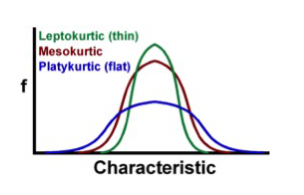Fig 5 Types of distribution curves

#### High positive correlation scatter graph

A scatterplot is a plot of paired (x, y) data with a horizontal x-axis and a vertical y-axis. Each individual pair is plotted as a single point. A scatter plot is used to visualise the relationship between two variables.

This graph implies that a person who scores high in Test X will also score high on Test Y. Note the slope is upward (as it moves to the right) indicating positivity. The more closely the plotted points fall on a straight line cutting the graph diagonally, the greater the correlation.Fig 6. High positive correlation scatter graph. Adapted from Pallant, J. (2013). SPSS survival manual: A step by step guide to data analysis using IBM SPSS (5th ed.). Sydney, Melbourne, Auckland, London: Allen & Unwin.

#### Minimal relationship scatter graph

There is no clear relationship between high and low, high and high and low and low scores in this graph.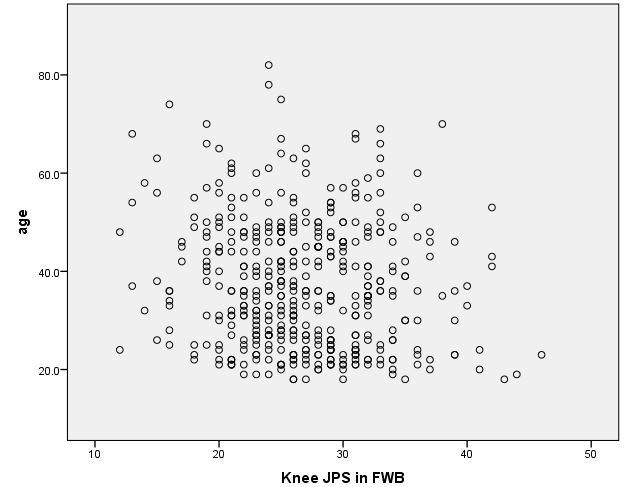Fig 7 Minimal relationship scatter graph. Adapted from Pallant, J. (2013). SPSS survival manual: A step by step guide to data analysis using IBM SPSS (5th ed.). Sydney, Melbourne, Auckland, London: Allen & Unwin.

#### High negative correlation scatter graph

This graph shows that a person who scores high in Test X will probably score low in Test Y. Similarly, those that score high in Test Y will score low in Test X. The slope of this graph is downwards (as it moves to the right) and indicates negativity. The more scattered the points are, the lower the correlation.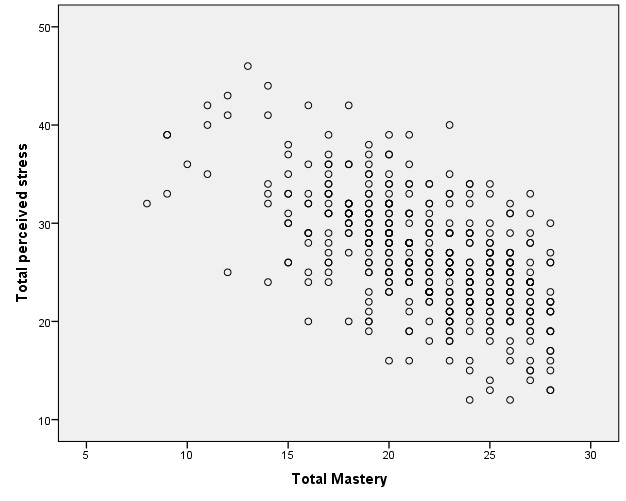Fig 8. High negative correlation scatter graph. Adapted from Pallant, J. (2013). SPSS survival manual: A step by step guide to data analysis using IBM SPSS (5th ed.). Sydney, Melbourne, Auckland, London: Allen & Unwin.

#### Statistical measurement used in allied health research

Many research papers use statistical measures such as: mean which summarises raw data that has been collected and organised when testing a hypothesis; standard deviation that shows variability from the mean; correlation coefficients that help measure possible relationships between variables that may be influencing the test results; risk ratios that measure the level of chance of something occurring or not; and the statistical significance testing or probability of the observed result being due to chance which uses p values.  These measurements may be demonstrated in Tables or by Graphs.

For more detailed information and resources, go to the Statistics page.

Mean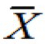is when all scores that have been collected are added together, then the total ( ∑x) is divided by the number of scores n, when x = a test score: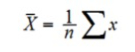Standard deviation is a measure of variability between groups that is comparable to the original measures obtained by using this formula s = √s2  when: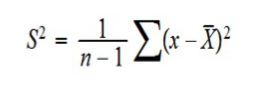Correlation coefficients are the numbers that indicate degree of relatedness between 2 or more variables.

0 means there is no correlation between the variables

-1 or +1 means maximum relationship between variables

-1 indicates a negative relationship

+1 indicates a positive relationship

Pearson product–moment  r is an example of a correlation coefficient. r is a measure of the relationship between 2 variables where when =1.00 means perfect correlation (but not necessary the cause) and where = 0 there is no correlation.

Coefficient of Determination is calculated using r and is the square of the correlation co-efficient. The result can be converted to % to explain to what extent the variability of one factor (eg IQ) is accounted for by the variability of another factor (eg Grade Point Average).

Cohen’s Rule of Thumb - Cohen’s d expresses the absolute change relative to the standard deviation. Calculate it by taking the absolute difference (mean difference between experimental and control group) and divide it by the standard deviation. This gives the SMD or Standardised mean difference or effect size.

SMD of 0.02 or less represents a small change

SMD of 0.50 represents a moderate change

SMD of 0.80 represents a large change

The t-test is an inferential statistic used to determine whether the means of two groups of scores differ to a statistically significant degree. It is used to test the null hypothesis, or that there is no difference in the means of the two groups.  The t-test is mainly applied to independent samples where subjects are assignment randomly to one group or another. A measurement of .05 or 5% is large enough to be statistically significant which means the null hypothesis can be rejected. This is written as p<.05.  One–tailed t-test/directional test is used when the direction of the difference is predicted before the data is collected. Two-tailed t-tests are used when there is some doubt about the results being significant prior to testing.

Risk ratios refer to the risk of an event occurring such as the odds of developing cancer from smoking

Values greater than 1.0 indicate increased risk.

Values less than 1.0 indicate reduced risk.

Values equal to 1.0 indicate the risk is no better than chance.

P values- Research scientists generally set the significance value for their experiments at 0.05, or 5 percent. This means that experimental results that meet this significance level have, at most, a 5% chance of being the result of pure chance. In other words, there's a 95% chance that the results were caused by the scientist's manipulation of experimental variables, rather than by chance. For most experiments, being 95% sure about a correlation between two variables is seen as "successfully" showing a correlation between the two. Put simply:

if the p<.05 the differences in results between cohorts are statistically significant and unlikely to have occurred by chance but if the p>.05 any difference is due to chance.

Ƒ – frequency

Raw data can be organised to show frequency of particular scores. The results can be arranged in a frequency distribution table or a graph. This shows how many times a particular score occurs.

#### Table 1. Frequency with raw data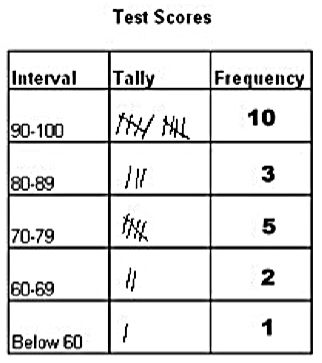Table 2. Frequency without raw dataTable 3.Frequency with NX – any value of the variable under consideration.

In the example this would be any interval of results e.g., 60-69.